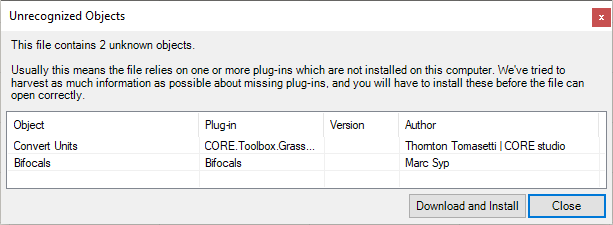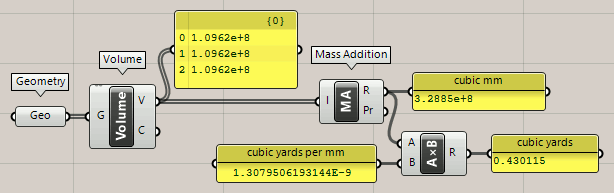# Convert Volume to Cubic Yards

How do we convert the Volume to cubic yards?

Convert mm to cu yrds.gh (11.1 KB)If your volume units are in cubic feetthen divide by 27 cubic feet per yard (3 * 3 * 3).

Oh wait, I see from the name of your GH file that you want to convert cubic millimeters to cubic yards?

1 mm^3 = 1.3079506193144E-9 yd^3
1 yd^3 = 764554857.984 mm^3

Example: convert 15 mm^3 to yd^3:
15 mm^3 = 15 × 1.3079506193144E-9 yd^3 = 1.9619259289716E-8 yd^3

So like this?Convert mm to cu yrds_2023Aug25a.gh (13.3 KB)

Advice: avoid passing component outputs through text panels.

@Joseph_Oster Thank you